# The pK, value for H2S is 7.00. What mole ratio of KHS to H2S is needed...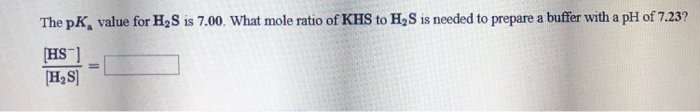The pK, value for H2S is 7.00. What mole ratio of KHS to H2S is needed to prepare a buffer with a pH of 7.23? HS H2S

Acidic buffer .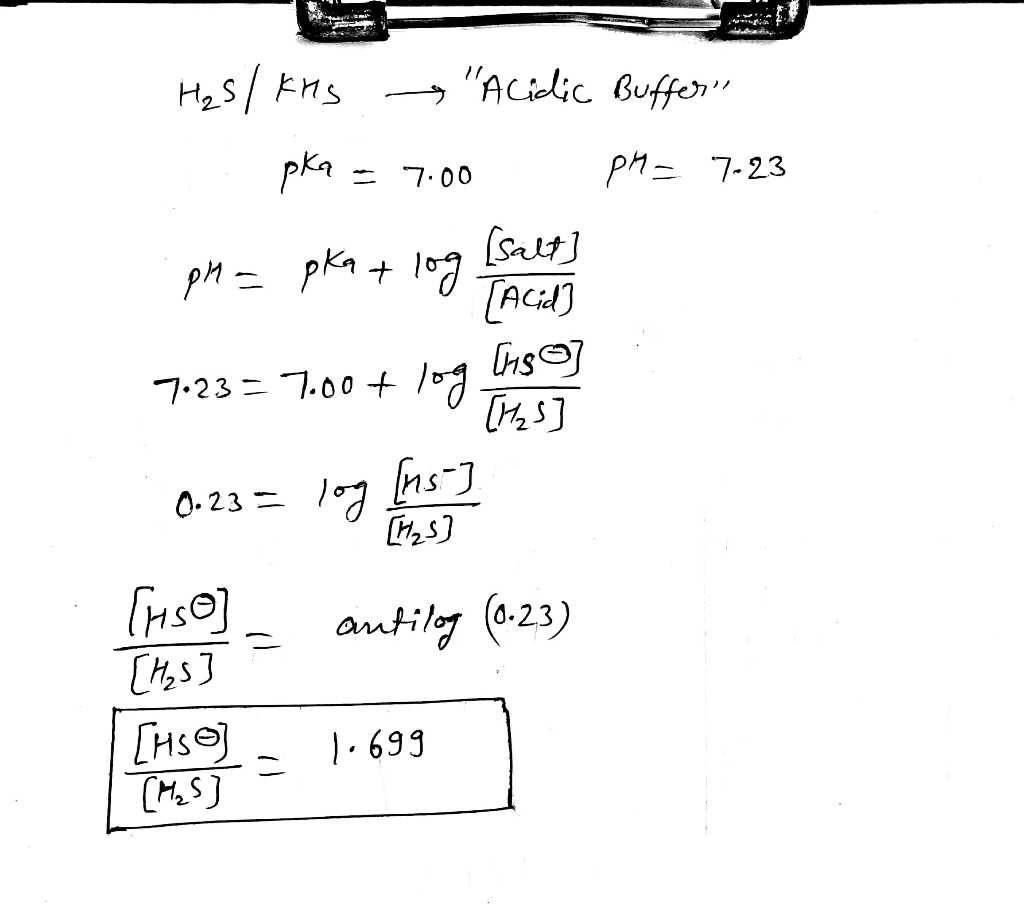#### Earn Coin

Coins can be redeemed for fabulous gifts.

Similar Homework Help Questions
• ### is 7.19. What mole ratio of K2 SO3 to KHSO3 is needed to prepare a buffer with a pH of 6.89? The pK value for HSO3 SO32...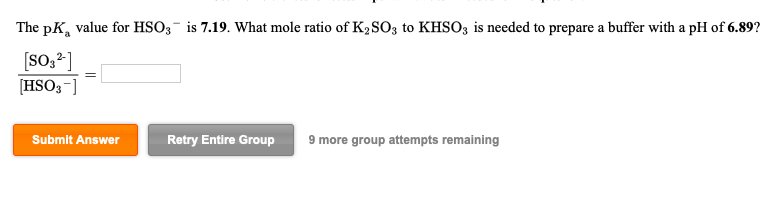is 7.19. What mole ratio of K2 SO3 to KHSO3 is needed to prepare a buffer with a pH of 6.89? The pK value for HSO3 SO32 HSO3] Submit Answer Retry Entire Group 9 more group attempts remaining is 7.19. What mole ratio of K2 SO3 to KHSO3 is needed to prepare a buffer with a pH of 6.89? The pK value for HSO3 SO32 HSO3] Submit Answer Retry Entire Group 9 more group attempts remaining

• ### a aTles if needed for this qsestion. p The pK, value for HC20 is 4.19. What...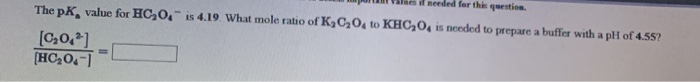a aTles if needed for this qsestion. p The pK, value for HC20 is 4.19. What mole ratio of K3C204 to KHC204 is needed to prepare a buffer with a pH of 4.55? [C204] HC,O4-

• ### A buffer solution is 0.419 M in H2S and 0.354 M in KHS. If Kg1 for...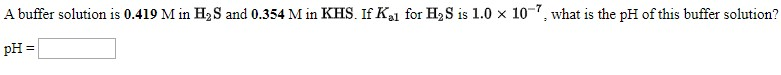A buffer solution is 0.419 M in H2S and 0.354 M in KHS. If Kg1 for H2S is 1.0 x 10-7, what is the pH of this buffer solution? pH =

• ### A buffer solution is made that is 0.491 M in H2S and 0.491 M in KHS...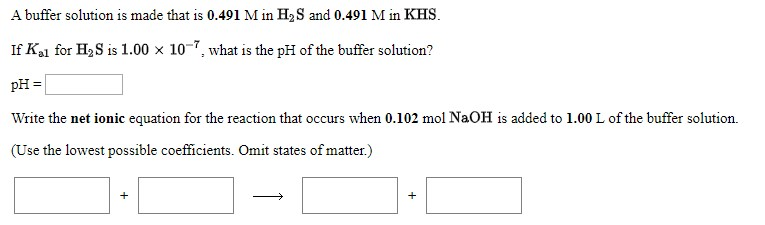A buffer solution is made that is 0.491 M in H2S and 0.491 M in KHS If Ka1 for H2S is 1.00 x 10, what is the pH of the buffer solution? pH= Write the net ionic equation for the reaction that occurs when 0.102 mol NaOH is added to 1.00 L of the buffer solution. (Use the lowest possible coefficients. Omit states of matter.)

• ### A buffer solution is made that is 0.342 M in H2S and 0.342 M in KHS....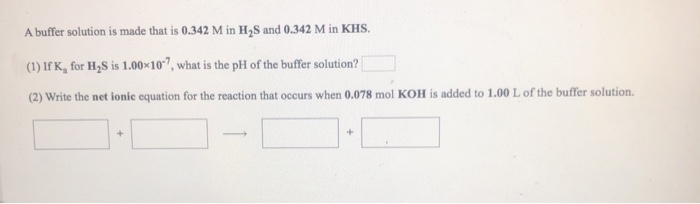A buffer solution is made that is 0.342 M in H2S and 0.342 M in KHS. (1) If K, for H2S is 1.0010-?, what is the pH of the buffer solution? (2) Write the net ionic equation for the reaction that occurs when 0.078 mol KOH is added to 1.00 L of the buffer solution. +

• ### A buffer solution is made that is 0.445 M in H2S and 0.445 M in KHS....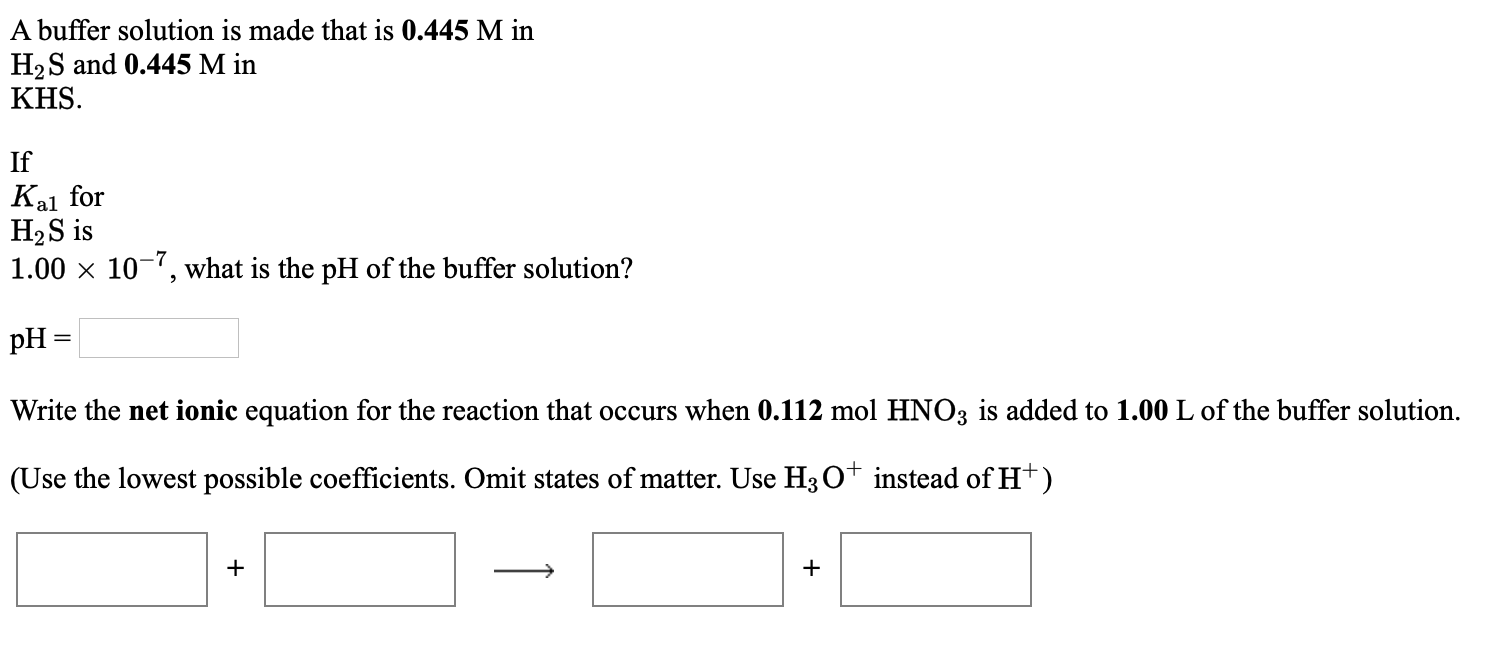A buffer solution is made that is 0.445 M in H2S and 0.445 M in KHS. Kal If for H2S is 1.00 x 10-7, what is the pH of the buffer solution? pH = Write the net ionic equation for the reaction that occurs when 0.112 mol HNO3 is added to 1.00 L of the buffer solution. (Use the lowest possible coefficients. Omit states of matter. Use H30+ instead of H+) + +

• ### A buffer solution is made that is 0.445 M in H2S and 0.445 M in KHS....A buffer solution is made that is 0.445 M in H2S and 0.445 M in KHS. Kal If for H2S is 1.00 x 10-7, what is the pH of the buffer solution? pH = Write the net ionic equation for the reaction that occurs when 0.112 mol HNO3 is added to 1.00 L of the buffer solution. (Use the lowest possible coefficients. Omit states of matter. Use H30+ instead of H+) + +

• ### A buffer solution is 0.475 M in H2S and 0.388 M in KHS. If K, for...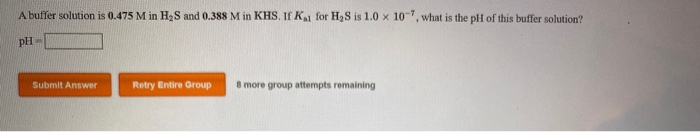A buffer solution is 0.475 M in H2S and 0.388 M in KHS. If K, for H, Sis 1.0 x 10-7, what is the pH of this buffer solution? pH Submit Answer Retry Entire Group 8 more group attempts remaining

• ### a) b) The pKa value for HCOs 10.10? is 10.30. What mole ratio of K2CO3 to...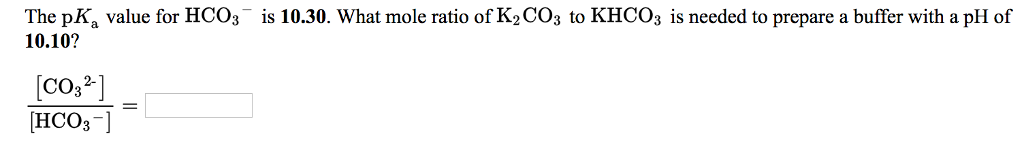a) b) The pKa value for HCOs 10.10? is 10.30. What mole ratio of K2CO3 to KHCOs is needed to prepare a buffer with a pH of CO,2 [HCO3-]

• ### A solution is prepared by adding 0.400 mol of hydrogen sulfide, H2S (pKa = 7.00) and...

A solution is prepared by adding 0.400 mol of hydrogen sulfide, H2S (pKa = 7.00) and 0.400 mol of the hydrogen sulfide ion, HS to 100.0 mL of water. Predict the final pH when 55.0 mL of 1.10 M NaOH is added to this buffer solution. Please explain. Thank you!